#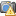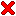Raytracing Special Relativityspeed of light in vacuum

To visualize the effects of special relativity, it takes a raytracer. When geometry is moving close to the speed of light with respect to the observer, the geometry appears to be curved: triangles, reflections, shadows... These non-linear effects are typically not captured by the routines of a standard graphics library.

Our raytracer uses the following conventions and supports the following features:

• the observer is at rest
• each triangle, and each light source moves with constant speed
• triangles carry texture coordinates, matt-, reflection-, and refraction coefficient
• a light source emmits from a single point
• the color of a light source may depend on (its own) time

The implementation of the project is guided by two aims:

• minimize the work necessary to setup interesting scenes
• optimize the performance of the raytracer

We combine different software: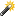Process of compilation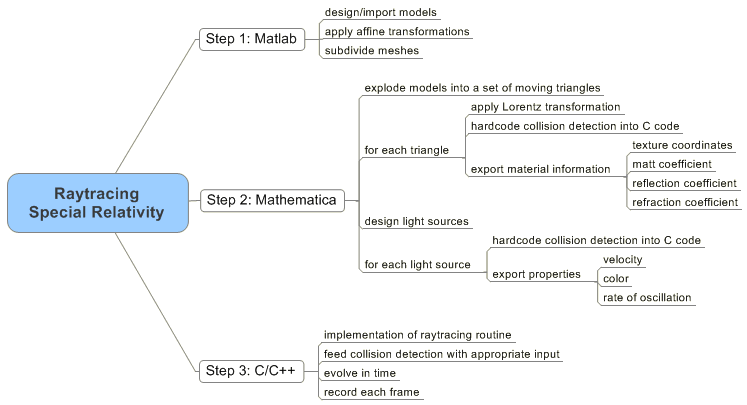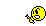Below, we show screen shots and animations of various scenes that were rendered by our raytracer. We briefly explain the setup of each scene. Then, we discuss all relevant mathematical issues that are involved in the implementation.

 Raytracing special relativity (sources, executable) *special_raytrace.zip 700 kB core source fileprog.cpp 20 kB
* includes Matlab, Mathematica, C++ code; executing the file prog.exe renders the animation two lenses behind glass.
It is a miracle that curiosity
survives formal education.
Albert Einstein

###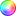Several examples

Click on the screenshots to view the full animations. Due to the compression applied in animated gif files, the original color palettes have been truncated. If not stated otherwise, the depth of recursion is 1 (which allows to trace one reflection, and refraction).flight through arch

The scene "flight through arch" aside demonstrates the effect, that straight lines appear curved.

There exist two light sources, which are at rest with respect to the observer. A violet light source to the left, and a white one more to the right.

The model of the Arch of Severus is adapted from greatbuildings.com. It has been reduced to 300 triangles in a rather unsophisticated manner.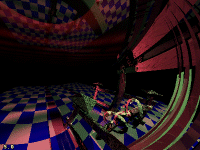hovercraft and Japanese gate

The scene "hovercraft and Japanese gate" comprises of 1000 triangles. Since the collision detection of the geometry is hardcoded, we reach the limit of the compiler. Total computation time of the 24 frames is about 3 hours.

The model of the Torii of Itsukushima is adapted from greatbuildings.com. The model of the hovercraft is from the Rice Comp460 class 2004. Unfortunately, the models are corrupt: double layered triangles, missing geometry.Earth passing by

Thomas Neukirchner helped me with physical aspects of reflection and refraction. But he also inspired me to do the rapid fly-by of planet Earth, which you see aside. Notice how the surface appears to roll. The three reflections show the Earth in the "past".

Erstaunlich finde ich aber immer wieder, dass die Kugel eine Kugel bleibt, was man bei Dir sehr schön sieht. Man kann sogar zeigen, dass sie nur konform verzerrt wird (Möbiustransformation). Daher waren mir Breiten- und Längenkreise wichtig, die bleiben nämlich immer senkrecht zueinander! TN

The trangulation of the sphere is adapted from a program by Darren Weber. The texture of our planet is adapted from Google Maps, which you can also view in high resolution.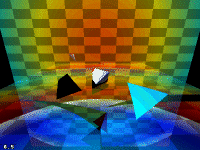tetrahedra, and time of objects

When objects move at different velocities, their watches appear to run asynchronous. In the animation aside, the evolution of color displays the object's evolution of time.

The objects in the middle are three tetrahedra, two of which are in motion. Notice the Doppler effect, how tetrahedra are approaching faster than moving away.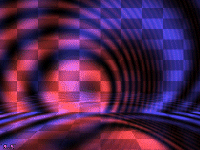American police car

A red light source moves to the right. A blue light source moves downwards. The intensity of both light sources oscillates.two lenses behind glass

For relativistic raytracing, refraction of rays is an interesting issue. The speed of light depends on the medium the ray is traversing. The refraction coefficient of a medium is the fraction of the speed of light in the medium relative to the speed of light in vacuum - see Snell's law.

In the scene aside, there are two flattened balls moving towards each other. In the front, there is a prism. The objects have different refraction coefficients. The total number of triangles is less than 200. Depth of recursion is 4.

The most beautiful thing we can experience is the mysterious.
It is the source of all true art and science.
Albert Einstein

###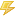Behind the scenes

In general, relativistic raytracing is similar to the non-relativistic scenario. We are just dealing with one additional dimension: time - of course. This allows us to take the speed of light into account, which contributes to the fact that we are always looking into the past.The core function of the raytracer shoot() traces a single ray. Ultimately, this function determines the color for each pixel on the screen. According to the depth of recursion, the function calls itself several times in order to trace reflections and refracted rays.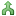The color of a pixel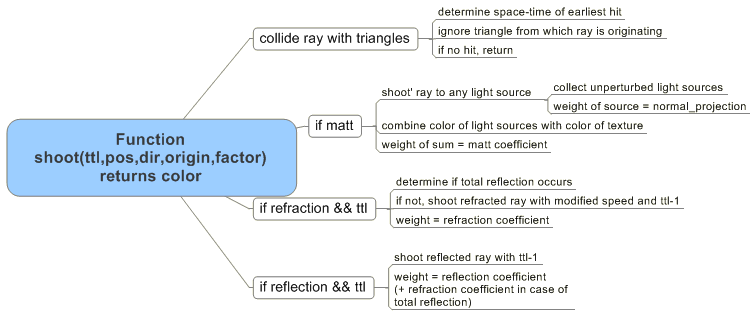Here comes the definition of the function shoot() in our C implementation:

```void shoot(int ttl,      // time to live (if equals 0 -> no recursive calls)
float *pos,   //              origin of ray in space-time
float *ray,   // direction and speed of ray in space-time
int origin,   // index of triangle to exclude from collision detection
float factor, // weight of color (prevents recursive calls if below threshold)
float *col    // returns RGB color
)
```

Let the coordinates of a triangle be known in rest space, say at time 0. If the triangle is moving with respect to the observer, the coordinates of the triangle undergo a linear transformation. The time component of a coordinate of the triangle might be different from zero after the linear transformation.

Assume a triangle with coordinates in space-time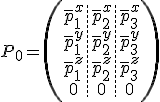is moving with speed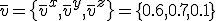with respect to an observer at rest. This is at the fractionof the speed of light. In general, relativity theory demands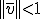. Now, using the space-time vectorthe coordinates of the triangle transform with respect to the observer asWe are interested in what is seen from the observer: Therefore, we shoot a light-like ray from the current position of observer into the past. To check if this ray hits a moving triangle, reduces to solve a system of four linear equations.

We assume, the position of the observer is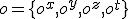. We shoot a ray in the past-like directionwith. Let the triangle initially be spanned by the three points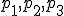in space time, which we gather in a matrix asWe assume, the triangle moves with speed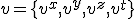. To detect if and when the ray hits the triangle in spacetime, we solve the equation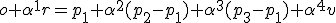The unknowns are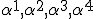. The variable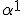is how much in the past we see the object. For a hit, we demand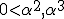, and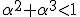. Then,are local parameters on the triangle, which are used for texture mapping. The ray hits the triangle at the pointNote, the equation above is equivalent to a system of linear equations, which we state in matrix notation asTo see a spot on a triangle, it has to be illuminated by a light source. Therefore, we need to determine the unique time when a ray is emitted from a light source so that it reaches the triangle in the right space-time. If the light source oscillates in intensity, this time is crucial.

Let the position of the light source at observer time t=0 be. We assume, that the light source moves with velocity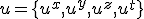with respect to the observer. We demand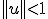.

We are interested in the observer time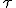when a ray from the light source reaches a given point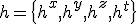in space time. This ray will travel in the direction of a light-like vector, thus the corresponding equation isor equivalently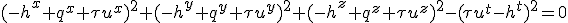The lhs is a quadratic polynomial in, and the equation usually has two solutions. For the application of raytracing, we are looking for the smaller value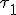.

Finally, we comment on reflection and refraction. The speed of light in vacuum is 299,792,458 m/s. The speed of light in air is decreased by a factor of 0.999707, in water by 0.750188, in glass by 0.666667, in salt by 0.647668, in diamond by 0.413394. However, these fractions vary slighly with the frequency.

We assume a light-like rayhits a surface normal to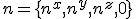. The angle of incidence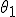is determined byA portion of the ray is reflected in the direction of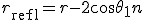Snell's law states that the ratio of the sines of the angles of incidence and refraction is equal to the ratio of velocities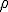in the two media. For instance,when going from water to glass. Depending on the sign of the scalar product, we define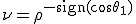. Given that, the angle of exit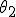is then determined by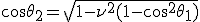otherwise, total reflection occurs. In vector notation, we yield the refracted ray asThe expressions above are valid only when the vectorsandare normalized to. However, to simulate a ray at a fraction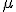of the speed of light in vacuum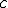, for instancefor diamond material, we perform raytracing with the scaled vectorMy grandfather once told me that there are two kinds of people:
Those who do the work and those who take the credit.
He told me to try to be in the first group;
there was much less competition.
Indira Gandhi